﻿ 面向林火持续侦察的多无人机分布式控制方法Download PDF文章快速检索 高级检索

Distributed control method of multiple UAVs for persistent wildfire surveillance
LIU Yuxuan, LIU Hu, TIAN Yongliang, SUN Cong
School of Aeronautic Science and Engineering, Beihang University, Beijing 100083, China
Abstract: In order to improve the practicality and autonomy in multiple UAV collaborative control for persistent wildfire surveillance, based on the Spread Vector Induced Celluar Automata (SVICA) fire spread algorithm, UAV kinematic, and sensor modeling, a more realistic three-dimension simulation environment for multiple UAV fire surveillance and the surveillance effectiveness indicators are constructed. A two-layer distributed control architecture for multiple UAV is proposed. Based on the reinforcement learning trained Artificial Neural Networks (ANN), the operational layer control realized the autonomous wildfire surrounding and terrain following under windy conditions. In the tactical layer, through the temporal even-distribution algorithm, the discrete airspeed adjustment of each UAV is performed to achieve the uniformity and immediacy of temporal distribution of UAVs in persistent wildfire surveillance. Then through a series of numerical experiments, the adaptability of the proposed distribution control method is verified under sudden UAV loss and supplement during the surveillance. In addition, based on the research on the relationship between the number of UAVs and the surveillance effectiveness, the UAV dispatch threshold is defined and enduring UAV dispatch and recycling strategy is examined. The final simulation results show that the proposed multiple UAV distributed control algorithm is effective and practical for persistent surveillance of wildfire.
Keywords: reinforcement learning    artificial neural network    multiple UAVs    distributed control    wildfire surveillance    fire spread simulation

1) 面向系统集成与实际运用，Martinez-de-Dios等[9-12]将视觉传感器、惯性导航和GPS等集成在现有无人机平台上，通过多次可控真实火场的实验，充分验证了多无人机林火侦察的技术可行性。此外，Ambrosia和Zajkowski验证了携带可见光相机低空短航时(Low-Altitude Short-Endurance，LASE)无人机与携带红外相机的中空长航时(Medium-Altitude Long-Endurance，MALE)无人机组合在林火信息收集中的作用。

2) 面向多无人机火场侦察协同控制策略，Casbeer等假设无人机只能在有限距离内同基站及其他无人机进行火场信息交换，以降低信息交换延迟为协同控制目标，控制无人机对(UAV pair)沿顺时针与逆时针按序出动。在此基础上，Alexis等假设无人机在汇合后进行折返，并基于火场信息共享与汇合点(rendezvous point)重计算，实现了多无人机在扩张火线上的动态分布。Ghamry和Zhang基于简化的椭圆火场蔓延模型构建无人机的参考路径，并使用引导-跟随(leader-follower)与滑模控制(Sliding Mode Control，SMC)方法，将多个四旋翼无人机均匀分布在预设的参考路径上。与此方法类似，Sujit等基于可扩张圆形火场假设，通过拍卖算法研究了多无人机多火点的分配问题，并通过扩张状态控制器使环绕圆形林火边界的无人机等距分布。Kumar等基于林火边界形状函数构建侦察效用函数，并通过该效用函数梯度下降、无人机间相互作用势函数与人工阻尼量生成每个无人机的控制向量，实现分布式的多无人机协同火场边界侦察。同样是势场控制(Potential Field Control，PFC)，Pham等基于无人机矩形下视场角模型构建了侦察代价函数，用于实现无人机自主火场覆盖与侦察。

1) 林火蔓延模型过分简化，大部分研究中火线几何形状过于理想化。部分算法需要火线准确的地理几何信息用于生成无人机参考路径或者人工势场。

2) 过分简化的飞行约束，上述二维平面的无人机协同控制算法没有考虑地形对无人机飞行控制的影响；没有根据林火热辐射等因素设置无人机安全飞行约束；没有考虑风速对无人机飞行的影响；并且忽略了烟雾对观测的遮挡作用。

3) 上述算法没有具体定义多无人火场信息侦察效能，并研究火线延长与所需无人机数量的匹配关系。随着无人机数量的增加，上述集中式多无人机控制算法往往具有较大计算成本。

1 无人机火场侦察建模 1.1 无人机控制模型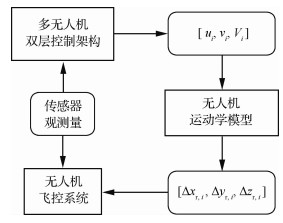图 1 无人机控制模型 Fig. 1 UAV control model

 $\left\{ {\begin{array}{*{20}{l}} {{{\dot x}_i} = {V_i}\cos {\varphi _i}\cos {\psi _i} + {v_{{\rm{w}}x}}}\\ {{{\dot y}_i} = {V_i}\sin {\varphi _i} + {v_{{\rm{w}}y}}}\\ {{{\dot z}_i} = {V_i}\cos {\varphi _i}\sin {\psi _i} + {v_{{\rm{w}}z}}} \end{array}} \right.$ （1）
 $\left\{ {\begin{array}{*{20}{l}} {{{\dot \varphi }_i} = \frac{{{\mathit{\Phi }_{\rm{u}}} + {\mathit{\Phi }_{\rm{d}}}}}{2} + {u_i}\frac{{{\mathit{\Phi }_{\rm{u}}} - {\mathit{\Phi }_{\rm{d}}}}}{2}}\\ {{{\dot \psi }_i} = \frac{{{\mathit{\Psi }_{\rm{l}}} + {\mathit{\Psi }_{\rm{r}}}}}{2} + {v_i}\frac{{{\mathit{\Psi }_{\rm{l}}} - {\mathit{\Psi }_{\rm{r}}}}}{2}} \end{array}} \right.$ （2）

 $\left\{ {\begin{array}{*{20}{l}} {{\varphi _{\min }} \le {\varphi _i} \le {\varphi _{\max }}}\\ {\sum\limits_{n = 1}^M {{V_i}} \left( {{t_n}} \right)\Delta t \le {L_{\max }}} \end{array}} \right.$ （3）

1.2 林火蔓延模型

 模型分类 优点 缺点 向量模型 火线边界无变形 缺乏林火属性，不适用平行计算 栅格模型 空间离散化，便于集成更多林火属性 火线边界变形 水平集模型 火线边界无变形 缺乏林火属性，火线必须封闭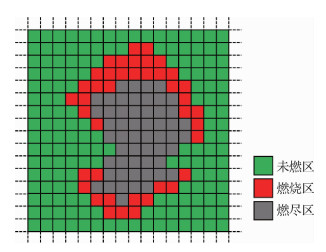图 2 林火栅格模型 Fig. 2 Raster-based representation of wildfire
1.3 林火侦察传感器模型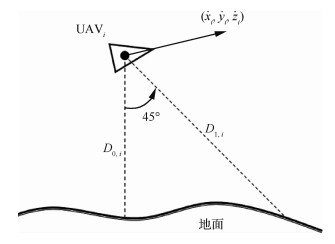图 3 对地测距传感器模型 Fig. 3 Model of downward ranging sensors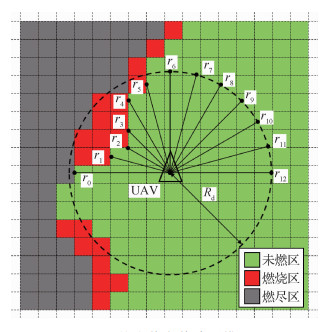图 4 林火侦察传感器模型 Fig. 4 Model of fire detection sensors
2 约束分析与问题描述

 $\left\{ {\begin{array}{*{20}{l}} {\left| {{D_{0,i}} - {H_{\rm{r}}}} \right| \le {H_{\rm{t}}}}\\ {{R_{\rm{d}}} \ge \min \left( {{R_i}} \right) \ge {R_{\rm{s}}}}\\ {\left| {\min \left( {{R_i}} \right) - {R_{\rm{r}}}} \right| \le {R_{\rm{t}}}} \end{array}} \right.$ （4）

 $\begin{array}{*{20}{l}} {{C_{{\rm{ff}}}} \buildrel \Delta \over = \left\{ {{C_{x,z}}\left| {{S_{x,z}} = 1} \right.} \right\} \cap }\\ {\left\{ {{C_{x,z}}|\exists i,j \in \{ - 1,0,1\} ,{S_{x + i,z + j}} = 0} \right\}} \end{array}$ （5）

 $\left\{ {\begin{array}{*{20}{l}} {E = \frac{1}{{card\left( {{C_{{\rm{ff}}}}} \right)}}\sum\limits_{{C_{x,z}} \in {C_{{\rm{ff}}}}} {{T_{x,z}}} }\\ {S = \sqrt {\frac{1}{{card\left( {{C_{{\rm{ff}}}}} \right)}}\sum\limits_{{C_{x,z}} \in {C_{{\rm{ff}}}}} {{{\left( {{T_{x,z}} - E} \right)}^2}} } }\\ {{T_{\max }} = \max \left( {\left\{ {{T_{x,z}}|{C_{x,z}} \in {C_{{\rm{ff}}}}} \right\}} \right)} \end{array}} \right.$ （6）

3 无人机双层控制设计 3.1 控制架构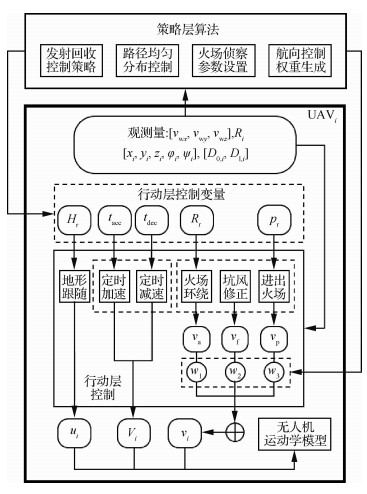图 5 多无人机双层控制架构 Fig. 5 Bi-level control framework of multipe UAVs

 ${v_i} = {w_1}{v_{\rm{a}}} + {w_2}{v_{\rm{f}}} + {w_3}{v_{\rm{p}}}$ （7）

3.2 行动层控制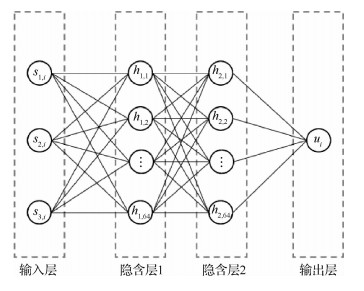图 6 地形跟随ANN结构 Fig. 6 ANN structure for terrain following
 $\left\{ {\begin{array}{*{20}{l}} {{s_{1,i}} = \frac{{2{D_{0,i}} - \sqrt 2 {D_{1,i}}}}{{2\sqrt {D_{0,i}^2 + D_{1,i}^2 - \sqrt 2 {D_{0,i}}{D_{1,i}}} }}}\\ {{s_{2,i}} = \sin {\varphi _i}}\\ {{s_{3,i}} = \frac{{{D_{0,i}} - {H_{\rm{r}}}}}{{{H_{\rm{r}}}}}} \end{array}} \right.$ （8）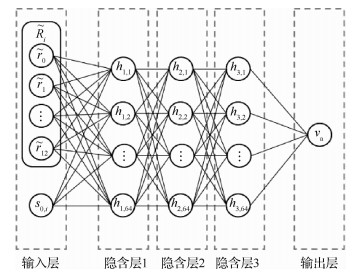图 7 火场环绕ANN结构 Fig. 7 ANN structure for wildfire surrounding
 ${s_{0,i}} = {\rm sgn}\left( {{r_0} - {r_{12}}} \right)$ （9）

 ${R_{\rm{u}}} = \left\{ {\begin{array}{*{20}{l}} { - 100}&{\left| {{D_{0,i}} - {H_{\rm{r}}}} \right| > {H_{\rm{t}}}}\\ { - 5}&{{H_{\rm{t}}} \ge \left| {{D_{0,i}} - {H_{\rm{r}}}} \right| > 5}\\ { - 1}&{5 \ge \left| {{D_{0,i}} - {H_{\rm{r}}}} \right| > 3}\\ { + 1}&{3 \ge \left| {{D_{0,i}} - {H_{\rm{r}}}} \right| > 1}\\ { + 10}&{1 \ge \left| {{D_{0,i}} - {H_{\rm{r}}}} \right| > 0.1}\\ { + 100}&{0.1 \ge \left| {{D_{0,i}} - {H_{\rm{r}}}} \right|} \end{array}} \right.$ （10）

 ${R_{\rm{v}}} = \left\{ {\begin{array}{*{20}{l}} { - 100}&{\min \left( {{R_i}} \right) < {R_{\rm{s}}}}\\ { - 1}&{{R_{\rm{r}}} - {R_{\rm{t}}} > \min \left( {{R_i}} \right) \ge {R_{\rm{s}}}}\\ { + 1}&{{R_{\rm{r}}} + {R_{\rm{t}}} \ge \min \left( {{R_i}} \right) \ge {R_{\rm{r}}} - {R_{\rm{t}}}}\\ { - 1}&{{R_{\rm{d}}} > \min \left( {{R_i}} \right) > {R_{\rm{r}}} + {R_{\rm{t}}}}\\ { - 50}&{\min \left( {{R_i}} \right) = {R_{\rm{d}}}} \end{array}} \right.$ （11）

 ${v_{\rm{f}}} = \left( {{v_{{\rm{w}}x}}\dot z - {v_{{\rm{w}}z}}\dot x} \right)/{V_{\rm{w}}}$ （12）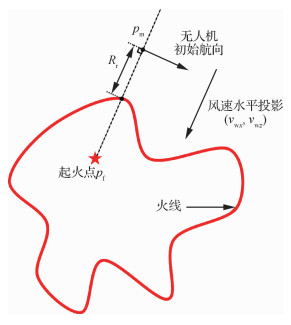图 8 进出火场任务区假设 Fig. 8 Mission zone entering and exiting assumptions

3.3 策略层设计

 ${X_i} = i\;\;\;\;i \in \{ 1,2, \cdots ,N\}$ （13）

 ${L_{{\rm{std}}{{\rm{,}}_i}}} = \frac{{{L_{1,{n_i}}}{L_{{\rm{c}},k}}}}{{{L_{{\rm{c}},1}}}}\;\;\;\;{L_{1,{n_i}}} \in {L_{{\rm{all}}}}$ （14）

 ${n_i} = \left\{ {\begin{array}{*{20}{l}} {\frac{{ {\rm card} \left( {{L_{{\rm{all}}}}} \right)\left( {{X_k} - {X_i}} \right)}}{N}}&{{X_i} \le {X_k}}\\ {\frac{{ {\rm card} \left( {{L_{{\rm{all}}}}} \right)\left( {N + {X_k} - {X_i}} \right)}}{N}}&{{X_i} > {X_k}} \end{array}} \right.$ （15）

 ${L_{{\rm{offset}}}} = {L_{{\rm{one}},1}} - {L_{{\rm{std}},1}}$ （16）

Lstd, iLone, i+Loffset≥0，则无人机UAVi当前里程相对理想里程滞后，加速时间tacc, i

 ${t_{{\rm{acc}},i}} = \frac{{{L_{{\rm{std}},i}} - {L_{{\rm{one}},i}} + {L_{{\rm{offset}}}}}}{{{V_{{\rm{max}}}} - {V_{\rm{c}}}}}$ （17）

 ${t_{{\rm{dec}},i}} = \frac{{{L_{{\rm{one}},i}} - {L_{{\rm{std}},i}} - {L_{{\rm{offset}}}}}}{{{V_{\rm{c}}} - {V_{{\rm{min}}}}}}$ （18）

 $\left( {{w_1},{w_2},{w_3}} \right) = \left\{ \begin{array}{l} \left( {{k_{\rm{a}}},1 - {k_{\rm{a}}},0} \right)\;\;\;\;\;火场环绕\\ \left( {0,1 - {k_{\rm{p}}},{k_{\rm{p}}}} \right)\;\;\;\;\;进出任务区 \end{array} \right.$ （19）

4 仿真实验与结果分析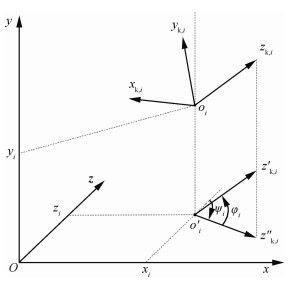图 9 Unity平台下坐标系说明 Fig. 9 Description of coordinate system in Unity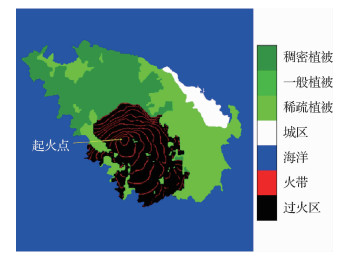图 10 SVICA林火蔓延仿真结果 Fig. 10 Simulation results of wildfire spread using SVICA model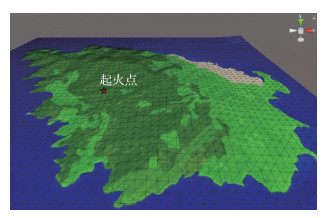图 11 Unity中斯佩特塞斯岛屿三维地形 Fig. 11 3D terrain of Spetses island in Unity

 参数符号 参数意义 数值 Vc/(m·s-1) 无人机巡航空速 30 Vmax/(m·s-1) 无人机最大空速 40 Vmin/(m·s-1) 无人机最小空速 20 Φu/((°)·s-1) 最大爬升角速度 60 Φd/((°)·s-1) 最小爬升角速度 -60 Ψr/((°)·s-1) 最大航向右转角速度 60 Ψl/((°)·s-1) 最大航向左转角速度 -60 Vw/(m·s-1) 风速 10 (vwx, vwy, vwz)/(m·s-1) 风速向量 (0, 0, -10) φmax/(°) 最大爬升角 30 φmin/(°) 最小爬升角 -30 Rd/m 有效探测距离 200 Rs/m 安全距离 40 Rt/m 距离浮动阈值 10 Rr/m 参考探测距离 100 Ht/m 高度浮动阈值 10 Hr/m 参考飞行真高 50 a/m 栅格尺寸 20 Lmax/km 最大飞行距离 50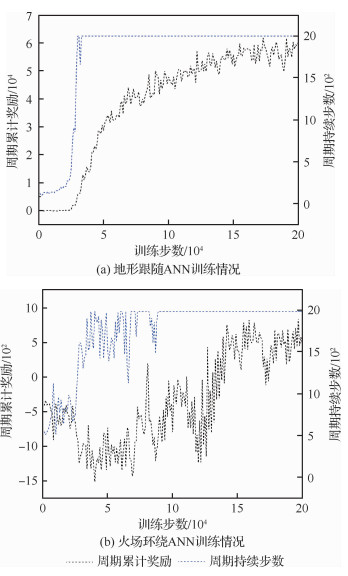图 12 ANN训练结果 Fig. 12 ANN training results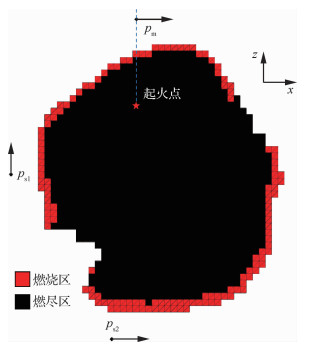图 13 ANN训练用仿真静态火场 Fig. 13 Static simulated wildfire for ANN training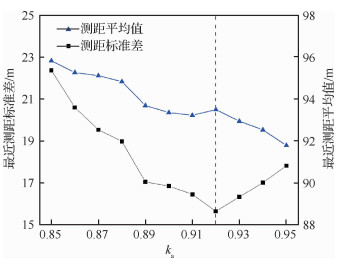图 14 不同ka对火场环绕的影响 Fig. 14 Effects on wildfire surrounding with different ka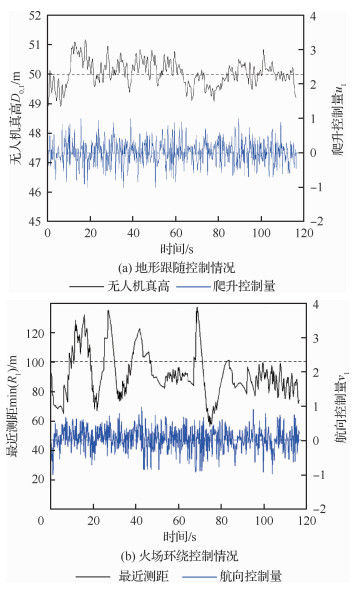图 15 无人机UAV1爬升和航向控制情况 Fig. 15 Climbing and heading control results of UAV1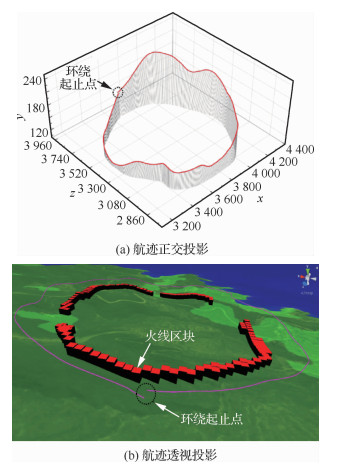图 16 无人机UAV1环绕火场第一圈航迹线 Fig. 16 Trajectory of UAV1 during the first wildfire surrounding cycle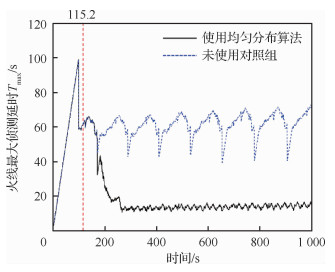图 17 效能指标Tmax的变化情况 Fig. 17 Evolution of the effectiveness indicatorTmax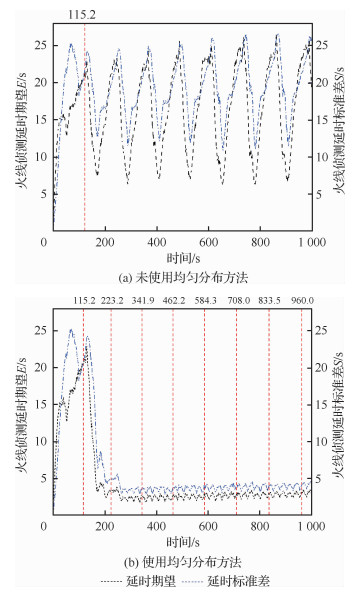图 18 效能指标E和S的变化情况 Fig. 18 Evolution of the effectiveness indicators E and S

2次实验分别取342~1 000 s的结果进行统计，可得使用均匀分布方法的5架无人机E的平均值为2.66 s，S的均值为3.78 s，Tmax的均值为13.84 s，分别远远小于未使用均匀分布方法E的平均值17.03 s、S的均值19.74 s以及Tmax的61.98 s。稳定且较小的ESTmax意味着，每个火线区块被无人机群以较小时间间隔均匀的探测，无人机群的火场侦察能力得到了较为充分的利用。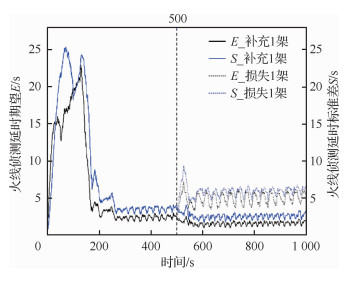图 19 无人机补充或损失情况下E和S的变化情况 Fig. 19 Evolution of E and S with UAV loss or replenishment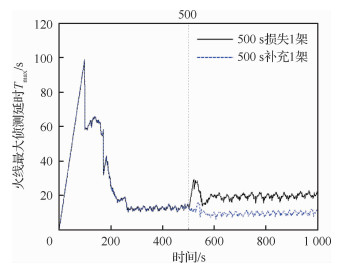图 20 无人机补充或损失情况下效能指标Tmax的变化情况 Fig. 20 Evolution of effectiveness indicator Tmax with UAV loss or replenishment

 无人机数量 效能指标 E/s S/s Tmax/s 1 44.80 32.54 105.93 2 18.14 15.61 49.32 3 9.13 9.26 29.70 4 4.92 5.86 19.85 5 2.73 3.86 14.02 6 1.55 2.52 10.10 7 0.90 1.61 7.34 8 0.55 0.99 5.28 9 0.36 0.57 3.66 10 0.26 0.30 2.36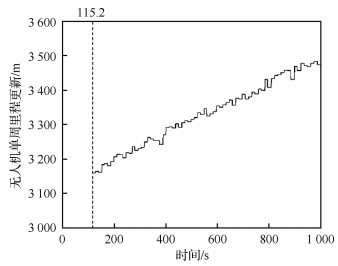图 21 10架UAV均匀分布方法单周标准里程变化 Fig. 21 Evolution of single-cycle trajectory length estimation with 10 UAVs using even-distributed method

 无人机数量 rcover/m 1 112.90 2 111.21 3 121.80 4 127.72 5 130.04 6 132.14 7 133.04 8 133.59 9 134.24 10 134.75

 ${{T'}_{\max }} = \frac{1}{{{V_{\rm{c}}}}}\left( {\frac{{{L_{{\rm{c}},i}}}}{N} - 2{{\bar r}_{{\rm{cover}}}}} \right)$ （20）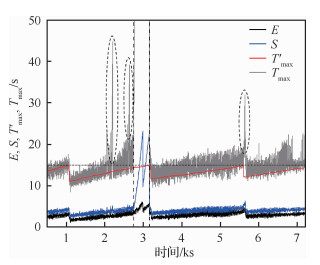图 22 2小时持续侦察测试结果 Fig. 22 Test results for two-hour persistent surveillance

5 结论

1) 在作者以往林火蔓延仿真研究的基础上，通过对无人机及传感器的建模，完成了较为复杂的仿真环境的构建。在此基础上，提出了多无人机持续火场侦察的效能指标。

2) 建立了多无人机分布式双层控制架构，并基于PPO强化学习算法完成了无人机行动层火场环绕与地形跟随两个ANN训练，并通过人工抗风修正方法实现了无人机基于局部侦察信息的自主火场环绕。在此基础上，提出了多无人机火场侦察时域均匀分布的速度调整方法，实现对无人机群在数量可变情况下，等时间间隔火场环绕的动态控制。

3) 通过一系列仿真实验与效能指标分析，本文提出的多无人机分布式控制方法得到充分的验证。在此基础上，通过对无人机数量与火场侦察效能的分析，提出了无人机出动阈值的概念，并通过长时间的仿真火场侦察实验，综合验证了本文提出的多无人机分布式控制架构在火场持续侦察任务中的可行性。

  TAO S, ZHANG L, CHEN W, et al. Mountains forest fire spread simulator based on geo-cellular automaton combined with Wang Zhengfei velocity model[J]. IEEE Journal of Selected Topics in Applied Earth Observations & Remote Sensing, 2013, 6(4): 1971-1987. Click to display the text  PHAM H X, LA H M, FEIL-SEIFER D, et al. A distributed control framework for a team of unmanned aerial vehicles for dynamic wildfire tracking[C]//2017 IEEE/RSJ International Conference on Intelligent Robots and Systems (IROS). Piscataway, NJ: IEEE Press, 2017: 6648-6653.  CASBEER D W, KINGSTON D B, BEARD R W, et al. Cooperative forest fire surveillance using a team of small unmanned air vehicles[J]. International Journal of Systems Science, 2006, 37(6): 351-360. Click to display the text  GHAMRY K A, ZHANG Y. Cooperative control of multiple UAVs for forest fire monitoring and detection[C]//2016 12th IEEE/ASME International Conference on Mechatronic and Embedded Systems and Applications (MESA). Piscataway, NJ: IEEE Press, 2016: 1-6.  YUAN C, ZHANG Y, LIU Z. A survey on technologies for automatic forest fire monitoring, detection, and fighting using unmanned aerial vehicles and remote sensing techniques[J]. Canadian Journal of Forest Research, 2015, 45(7): 783-792. Click to display the text  SUJIT P B, KINGSTON D, BEARD R. Cooperative forest fire monitoring using multiple UAVs[C]//2007 46th IEEE Conference on Decision and Control. Piscataway, NJ: IEEE Press, 2007: 4875-4880.  AMBROSIA V G, ZAJKOWSKI T. Selection of appropriate class UAS/sensors to support fire monitoring:Experiences in the United States[J]. Handbook of Unmanned Aerial Vehicles, 2015, 2723-2754. Click to display the text  AKHLOUFI M A, CASTRO N A, COUTURIER A. UAVs for wildland fires[C]//Autonomous Systems: Sensors, Vehicles, Security, and the Internet of Everything, 2018.  MARTÍNEZ-DE-DIOS J R, MERINO L, OLLERO A, et al. Multiple heterogeneous unmanned aerial vehicles[M]. Berlin: Springer, 2007: 207-228.  MERINO L, CABALLERO F, MARTÍNEZ-DE-DIOS J R, et al. Automatic forest fire monitoring and measurement using unmanned aerial vehicles[C]//Proceedings of the 6th International Congress on Forest Fire Research, 2010.  MERINO L, CABALLERO F, MARTÍNEZ-DE-DIOS J R, et al. An unmanned aircraft system for automatic forest fire monitoring and measurement[J]. Journal of Intelligent & Robotic Systems, 2012, 65(1-4): 533-548. Click to display the text  MERINO L, MARTÍNEZ-DE DIOS J R, OLLERO A. Cooperative unmanned aerial systems for fire detection, monitoring, and extinguishing[M]//Handbook of Unmanned Aerial Vehicles. Dordrecht:Springer, 2015:2693-2722.  AMBROSIA V G, ZAJKOWSKI T. Selection of appropriate class UAS/sensors to support fire monitoring:experiences in the United States[M]//Handbook of Unmanned Aerial Vehicles. Dordrecht:Springer, 2015:2723-2754.  ALEXIS K, NIKOLAKOPOULOS G, TZES A, et al. Applications of intelligent control to engineering systems[M]. Dordrecht: Springer, 2009.  KUMAR M, COHEN K, HOMCHAUDHURI B. Cooperative control of multiple uninhabited aerial vehicles for monitoring and fighting wildfires[J]. Journal of Aerospace Computing, Information, and Communication, 2011, 8(1): 1-16. Click to display the text  王祝, 刘莉, 龙腾, 等. 基于罚函数序列凸规划的多无人机轨迹规划[J]. 航空学报, 2016, 37(10): 3149-3158. WANG Z, LIU L, LONG T, et al. Trajectory planning for multi-UAVs using penalty sequential convex programming[J]. Acta Aeronautica et Astronautica Sinica, 2016, 37(10): 3149-3158. (in Chinese) Cited By in Cnki | Click to display the text  YAO P, WANG H, SU Z. Cooperative path planning with applications to target tracking and obstacle avoidance for multi-UAVs[J]. Aerospace Science and Technology, 2016, 54: 10-22. Click to display the text  SULLIVAN A L. Wildland surface fire spread modelling, 1990-2007.3:Simulation and mathematical analogue models[J]. International Journal of Wildland Fire, 2009, 18(4): 387-403. Click to display the text  LIU Y, LIU H, ZHOU Y, et al. Spread vector induced cellular automata model for real-time crown fire behavior simulation[J]. Environmental Modelling & Software, 2018, 108: 14-39. Click to display the text  MARTÍNEZ-DE DIOS J, MERINO L, CABALLERO F, et al. Automatic forest-fire measuring using ground stations and unmanned aerial systems[J]. Sensors, 2011, 11(6): 6328-6353. Click to display the text  RAMACHANDRAN P, ZOPH B, LE Q V. Searching for activation functions[EB/OL]. (2017-10-27)[2019-08-06]. https://arxiv.org/abs/1710.05941.  SCHULMAN J, WOLSKI F, DHARIWAL P, et al. Proximal policy optimization algorithms[EB/OL]. (2017-08-28)[2019-08-06]. https://arxiv.org/abs/1707.06347.  LUGO-CARDENAS I, FLORES G, SALAZAR S, et al. Dubins path generation for a fixed wing UAV[C]//2014 International conference on unmanned aircraft systems (ICUAS). Piscataway, NJ: IEEE Press, 2014: 339-346.  Unity Technologies. Unity[EB/OL].[2019-08-06]. http://unity3d.com.  JULIANI A, BERGES V, VCKAY E, et al. Unity: A general platform for intelligent agents[EB/OL]. (2018-09-07)[2019-08-06]. https://arxiv.org/abs/1809.02627.
http://dx.doi.org/10.7527/S1000-6893.2019.23381

0

#### 文章信息

LIU Yuxuan, LIU Hu, TIAN Yongliang, SUN Cong

Distributed control method of multiple UAVs for persistent wildfire surveillance

Acta Aeronautica et Astronautica Sinica, 2020, 41(2): 323381.
http://dx.doi.org/10.7527/S1000-6893.2019.23381